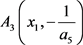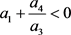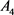# 一类三次多项式系统的定性分析Qualitative Analysis of a Class of Cubic Polynomial Systems

• 全文下载: PDF(502KB)    PP.205-212   DOI: 10.12677/DSC.2019.83022
• 下载量: 283  浏览量: 365   科研立项经费支持

In this paper, the classical method of qualitative analysis is used to analyze the existence, type and local stability of a class of planar cubic polynomial differential system dx/dt=-y+a1x+a2x2+a3y3+a4xy2dy/dt=x(1+a5y). And the formal series method is used to determine the center-focus of the singular point. Finally, the conditions of the existence of limit cycles of the system are obtained by using the Hopf bifurcation method.

1. 引言(1)

2. 预备知识

2.1. 奇点定义  

2.2. 奇点的类型  

2.3. Hopf分支问题  

$\left\{\begin{array}{l}\frac{\text{d}x}{\text{d}t}=P\left(x,y,\lambda \right)\\ \frac{\text{d}y}{\text{d}t}=Q\left(x,y,\lambda \right)\end{array}$ (2)

3. 奇点分析

$\left\{\begin{array}{l}-y+{a}_{1}x+{a}_{2}{x}^{2}+{a}_{3}{y}^{3}+{a}_{4}x{y}^{2}=0\\ x\left(1+{a}_{5}y\right)=0\end{array}$ (3)

2) 若 ${a}_{3}>0$ ，则系统(1)有奇点 ${A}_{1}\left(0,{y}_{0}\right)$${A}_{2}\left(0,-{y}_{0}\right)$ ，其中 ${y}_{0}=\frac{1}{\sqrt{{a}_{3}}}$

3) 若 ${a}_{5}\ne 0$ ，则系统(1)有奇点${A}_{4}\left({x}_{2},-\frac{1}{{a}_{5}}\right)$ ，其中 ${x}_{1}=\frac{-\left({a}_{1}+\frac{{a}_{4}}{{a}_{5}^{2}}\right)+\sqrt{\Delta }}{2{a}_{2}}$${x}_{2}=\frac{-\left({a}_{1}+\frac{{a}_{4}}{{a}_{5}^{2}}\right)-\sqrt{\Delta }}{2{a}_{2}}$$\Delta ={\left({a}_{1}+\frac{{a}_{4}}{{a}_{5}^{2}}\right)}^{2}-4{a}_{2}\left(\frac{1}{{a}_{5}}-\frac{{a}_{3}}{{a}_{5}^{3}}\right)$

3.1. 奇点 $O\left(0,0\right)$

2) 当 ${a}_{1}\le -2$$O\left(0,0\right)$ 为系统(1)的稳定结点， ${a}_{1}\ge 2$ 时为不稳定结点。

3) 当 ${a}_{1}=0$$O\left(0,0\right)$ 为系统(1)的中心型奇点(中心或者细焦点)。

3.2. 奇点 ${A}_{1}\left(0,{y}_{0}\right)$

2) 若 ${a}_{5}>-\sqrt{{a}_{3}}$ ，则特征根为异号实根， ${A}_{1}\left(0,{y}_{0}\right)$ 为系统(1)的鞍点。

3) 若 ${a}_{5}<-\sqrt{{a}_{3}}$ ，则

i) 当 ${\left({a}_{1}+\frac{{a}_{4}}{{a}_{3}}\right)}^{2}+8\left(1+\frac{{a}_{5}}{\sqrt{{a}_{3}}}\right)>0$ 时特征根为同号实根， ${A}_{1}\left(0,{y}_{0}\right)$ 为系统(1)的结点， ${a}_{1}+\frac{{a}_{4}}{{a}_{3}}>0$ 时不稳定， ${a}_{1}+\frac{{a}_{4}}{{a}_{3}}<0$ 时稳定。

ii) 当 ${\left({a}_{1}+\frac{{a}_{4}}{{a}_{3}}\right)}^{2}+8\left(1+\frac{{a}_{5}}{\sqrt{{a}_{3}}}\right)<0$ 时特征根为共轭复根， ${A}_{1}\left(0,{y}_{0}\right)$ 为系统(1)的焦点， ${a}_{1}+\frac{{a}_{4}}{{a}_{3}}>0$ 时不稳定， ${a}_{1}+\frac{{a}_{4}}{{a}_{3}}<0$ 时稳定。 ${a}_{1}+\frac{{a}_{4}}{{a}_{3}}=0$${A}_{1}\left(0,{y}_{0}\right)$ 为系统(1)的中心型奇点(中心或者细焦点)。

3.3. 奇点 ${A}_{2}\left(0,-{y}_{0}\right)$

2) 若 ${a}_{5}<\sqrt{{a}_{3}}$ ，则特征根为异号实根， ${A}_{2}\left(0,-{y}_{0}\right)$ 为系统(1)的鞍点。

3) 若 ${a}_{5}>\sqrt{{a}_{3}}$ ，则

i) 当 ${\left({a}_{1}+\frac{{a}_{4}}{{a}_{3}}\right)}^{2}+8\left(1+\frac{{a}_{5}}{\sqrt{{a}_{3}}}\right)>0$ 时特征根为同号实根， ${A}_{2}\left(0,-{y}_{0}\right)$ 为系统(1)的结点， ${a}_{1}+\frac{{a}_{4}}{{a}_{3}}>0$ 时不稳定， ${a}_{1}+\frac{{a}_{4}}{{a}_{3}}<0$ 时稳定。

ii) 当 ${\left({a}_{1}+\frac{{a}_{4}}{{a}_{3}}\right)}^{2}+8\left(1+\frac{{a}_{5}}{\sqrt{{a}_{3}}}\right)<0$ 时特征根为共轭复根， ${A}_{2}\left(0,-{y}_{0}\right)$ 为系统(1)的焦点， ${a}_{1}+\frac{{a}_{4}}{{a}_{3}}>0$ 时不稳定，时稳定。 ${a}_{1}+\frac{{a}_{4}}{{a}_{3}}=0$${A}_{2}\left(0,-{y}_{0}\right)$ 为系统(1)的中心型奇点(中心或者细焦点)。

3.4. 奇点 ${A}_{3}\left({x}_{1},-\frac{1}{{a}_{5}}\right)$

2) 当 ${d}_{2}<0$ 时， ${A}_{3}$ 为系统的鞍点。

3) 若 ${d}_{2}>0$ ，则

i) 当 ${d}_{1}^{2}-4{d}_{2}\ge 0$ 时，特征根为同号实根， ${A}_{3}$ 为系统(1)的结点， ${d}_{1}>0$ 时不稳定， ${d}_{1}<0$ 时稳定。

ii) 若 ${d}_{1}^{2}-4{d}_{2}<0$ 时，特征根为共轭复根， ${A}_{3}$ 为系统(1)的焦点， ${d}_{1}>0$ 时不稳定， ${d}_{1}<0$ 时稳定。 ${d}_{1}=0$${A}_{3}$ 为系统(1)的中心型奇点(中心或者细焦点)。

3.5. 奇点 ${A}_{4}\left({x}_{2},-\frac{1}{{a}_{5}}\right)$

2) 当 ${d}_{4}<0$ 时， ${A}_{4}$ 为系统的鞍点。

3) 若 ${d}_{4}>0$ ，则

i) 当 ${d}_{3}^{2}-4{d}_{4}\ge 0$ 时，特征根为同号实根， ${A}_{4}$ 为系统(1)的结点， ${d}_{3}>0$ 时不稳定， ${d}_{3}<0$ 时稳定。

ii) 若 ${d}_{3}^{2}-4{d}_{4}<0$ 时，特征根为共轭复根，为系统(1)的焦点， ${d}_{3}>0$ 时不稳定，时稳定。 ${d}_{3}=0$${A}_{4}$ 为系统(1)的中心型奇点(中心或者细焦点)。

3.6. 细焦点

$\left\{\begin{array}{l}\frac{\text{d}y}{\text{d}t}=-y+{a}_{2}{x}^{2}+{a}_{3}{y}^{3}+{a}_{4}x{y}^{2}\\ \frac{\text{d}y}{\text{d}t}=x+{a}_{5}xy\end{array}$ (4)

2) 当 ${a}_{4}<0$ 时， $O\left(0,0\right)$ 是系统(4)的一阶稳定细焦点。

3) 当 ${a}_{4}=0$ 时， $O\left(0,0\right)$ 为中心。

$\begin{array}{c}{\frac{\text{d}F}{\text{d}t}|}_{\left(1\right)}=\frac{\partial F}{\partial x}\stackrel{˙}{x}+\frac{\partial F}{\partial y}\stackrel{˙}{y}\\ =\left(2x+\frac{\partial {F}_{3}}{\partial x}+\frac{\partial {F}_{4}}{\partial x}+\cdots \right)\left(-y+{a}_{2}{x}^{2}+{a}_{3}{y}^{3}+{a}_{4}x{y}^{2}\right)\\ \text{\hspace{0.17em}}\text{\hspace{0.17em}}+\left(2y+\frac{\partial {F}_{3}}{\partial y}+\frac{\partial {F}_{4}}{\partial y}+\cdots \right)\left(x+{a}_{5}xy\right)\end{array}$ (5)

$\frac{\text{d}{F}_{3}}{\text{d}\theta }=-2\left({a}_{2}{\mathrm{cos}}^{3}\theta +{a}_{5}\mathrm{cos}\theta {\mathrm{sin}}^{2}\theta \right)$ (6)

${\int }_{0}^{2\text{π}}{a}_{3}{\mathrm{cos}}^{3}\theta +{a}_{5}\mathrm{cos}\theta {\mathrm{sin}}^{2}\theta =0$ ，故对(6)式两边进行积分得：

${F}_{3}\left(\mathrm{cos}\theta ,\mathrm{sin}\theta \right)=-\frac{4}{3}{a}_{2}{\mathrm{sin}}^{3}\theta -\frac{2}{3}{a}_{5}{\mathrm{sin}}^{3}\theta -2{a}_{2}\mathrm{sin}\theta {\mathrm{cos}}^{2}\theta$ ，即 ${F}_{3}\left(x,y\right)=-\frac{4}{3}{a}_{2}{y}^{3}-2{a}_{2}{x}^{2}y-\frac{2}{3}{a}_{5}{y}^{3}$

$2{a}_{3}x{y}^{3}+2{a}_{4}{x}^{2}{y}^{2}+{a}_{2}{x}^{2}\frac{\partial {F}_{3}}{\partial x}-y\frac{\partial {F}_{4}}{\partial x}+{a}_{5}xy\frac{\partial {F}_{3}}{\partial y}+x\frac{\partial {F}_{4}}{\partial y}=0$

$\left(2{a}_{3}-4{a}_{2}{a}_{5}-2{a}_{5}^{2}\right)x{y}^{3}-\left(4{a}_{2}^{2}+2{a}_{2}{a}_{5}\right){x}^{3}y+2{a}_{4}{x}^{2}{y}^{2}+x\frac{\partial {F}_{4}}{\partial y}-y\frac{\partial {F}_{4}}{\partial x}=0$ ，将上式取极坐标 $x=r\mathrm{cos}\theta$$y=r\mathrm{sin}\theta$ 并消去 ${r}^{4}$ 得：

$\frac{\text{d}{F}_{4}}{\text{d}\theta }=\left(4{a}_{2}{a}_{5}+2{a}_{5}^{2}-2{a}_{3}\right)\mathrm{cos}\theta {\mathrm{sin}}^{3}\theta +\left(2{a}_{2}{a}_{5}+4{a}_{2}^{2}\right){\mathrm{cos}}^{3}\theta \mathrm{sin}\theta -2{a}_{4}{\mathrm{cos}}^{2}\theta {\mathrm{sin}}^{2}\theta =-{H}_{4}\left(\mathrm{cos}\theta ,\mathrm{sin}\theta \right)$

${a}_{4}\ne 0$ 时， ${\int }_{0}^{2\text{π}}{H}_{4}\left(\mathrm{cos}\theta ,\mathrm{sin}\theta \right)\text{d}\theta =2{a}_{4}{\int }_{0}^{\text{2π}}{\mathrm{cos}}^{2}\theta {\mathrm{sin}}^{2}\theta \text{d}\theta =\frac{{a}_{4}\text{π}}{2}\ne 0$ ，因此当 ${a}_{4}\ne 0$ 时， ${C}_{4}=\frac{1}{\text{2π}}{\int }_{0}^{\text{2π}}{H}_{4}\left(\mathrm{cos}\theta ,\mathrm{sin}\theta \right)\text{d}\theta =\frac{{a}_{4}}{4}\ne 0$ ，且 ${C}_{4}$${a}_{4}$ 同号。

$\phi \left(x,y\right)={x}^{2}+{y}^{2}+{F}_{3}+{F}_{4}$ ，那么 ${\frac{\text{d}\phi }{\text{d}t}|}_{\left(1\right)}={r}^{4}{C}_{4}+ο\left({r}^{4}\right)$ ，进而有结论：

1) 当 ${a}_{4}>0$ 时， $O\left(0,0\right)$ 是系统(1)的不稳定细焦点。

2) 当 ${a}_{4}<0$ 时， $O\left(0,0\right)$ 是系统(1)的稳定细焦点。

${a}_{4}=0$ 时，因 $P\left(-x,y\right)=P\left(x,y\right)$$Q\left(-x,y\right)=-Q\left(x,y\right)$ ，由对称原理得 $O\left(0,0\right)$ 为中心。

4. 极限环的存在性

1) ${a}_{4}>0$${a}_{1}<0$$|{a}_{1}|\ll 1$

2) ${a}_{4}<0$$0<{a}_{1}\ll 2$

${a}_{4}<0$${a}_{1}=0$$O\left(0,0\right)$ 为稳定的细焦点，而当 $0<{a}_{1}<2$ 时系统(1)以 $O\left(0,0\right)$ 为不稳定焦点。由Hopf分支问题的Liapunov第二方法可知在条件(2)下，系统(1)在奇点外至少产生一个稳定极限环。

  秦元勋. 学习H. Poincare的数学工作思想方法[J]. 西北大学学报, 1982(1): 1-7.  Poincaré, H. (1881) Mémoire sur les courbes définie par une équation differentielle. Journal de mathématiques pures et appliquées, 7, 375-422.  Hilbert, D. (1901) Mathematische Probleme. Archiv der Mathematik und Physik, 1, 44-63, 213-237.  Dulac, H. (1923) Sur les Cycles Limites. Bulletin de la Société Mathématique de France, 51, 45-188. https://doi.org/10.24033/bsmf.1031  史松龄. 二次系统(E2)出现至少四个极限环的例子[J]. 中国科学, 1979(11): 1051-1056.  陈兰荪, 王明淑. 二次系统极限环的相对位置与个数[J]. 数学学报, 1979, 22(6): 751-758.  叶彦谦. 极限环论[M]. 上海: 上海科学技术出版社, 1984.  叶彦谦. 多项式微分系统定性理论[M]. 上海: 上海科学技术出版, 1995.  卜令杰, 窦霁虹, 刘萌萌, 等. 一类三次系统极限环的存在唯一性[J]. 延安大学学报(自然科学版), 2014, 33(2): 1-5.  朱科科. 一类三次系统极限环的存在性[J]. 安阳师范学院学报, 2017(5): 4-8.  马知恩. 一类三次系统极限环的存在唯一性[J]. 数学年刊A辑(中文版), 1999, 19(1): 16-18.  周久红. 一类三次系统的定性分析[D]: [硕士学位论文]. 合肥: 安徽大学, 2013.  东北师范大学微分方程教研室. 常微分方程[M]. 北京: 高等教育出版社, 2005.  丁同仁, 李承治. 常微分方程教程[M]. 第2版. 北京: 高等教育出版社, 2004.  张锦炎. 常微分方程几何理论与分支问题[M]. 北京: 北京大学出版社, 1981.  罗定军, 张祥, 董梅芳. 动力系统的定性与分支理论[M]. 北京: 科学出版社, 2001.  张芷芬, 丁同仁, 黄文灶, 董镇喜. 微分方程定性理论[M]. 北京: 科学出版社, 1985.  Luo, D., Wang, X., Zhu, D. and Han, M. (1997) Bifurcation Theory and Methods of Dynamical Systems. In: Advanced Series in Dynamical Systems, vol. 15, World Scientific, Singapore. https://doi.org/10.1142/2598